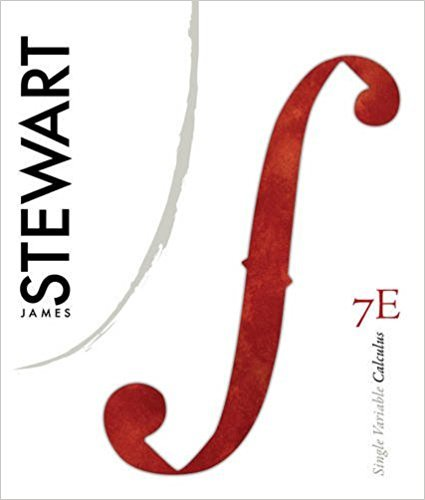×
Get Full Access to Single Variable Calculus - 7 Edition - Chapter 4 - Problem 50
Get Full Access to Single Variable Calculus - 7 Edition - Chapter 4 - Problem 50

×

# Solved: The Fresnel function was introduced in SectionISBN: 9780538497831 151

## Solution for problem 50 Chapter 4

Single Variable Calculus | 7th Edition

• Textbook Solutions
• 2901 Step-by-step solutions solved by professors and subject experts
• Get 24/7 help from StudySoup virtual teaching assistantsSingle Variable Calculus | 7th Edition

4 5 1 328 Reviews
24
2
Problem 50

The Fresnel function was introduced in Section 4.3. Fresnel also used the function in his theory of the diffraction of light waves. (a) On what intervals is increasing? (b) On what intervals is concave upward? (c) Use a graph to solve the following equation correct to two decimal places: (d) Plot the graphs of and on the same screen. How are these graphs related

Step-by-Step Solution:
Step 1 of 3

Step 2 of 3

Step 3 of 3

##### ISBN: 9780538497831

Since the solution to 50 from 4 chapter was answered, more than 260 students have viewed the full step-by-step answer. Single Variable Calculus was written by and is associated to the ISBN: 9780538497831. The full step-by-step solution to problem: 50 from chapter: 4 was answered by , our top Calculus solution expert on 11/15/17, 04:21PM. The answer to “The Fresnel function was introduced in Section 4.3. Fresnel also used the function in his theory of the diffraction of light waves. (a) On what intervals is increasing? (b) On what intervals is concave upward? (c) Use a graph to solve the following equation correct to two decimal places: (d) Plot the graphs of and on the same screen. How are these graphs related” is broken down into a number of easy to follow steps, and 64 words. This full solution covers the following key subjects: fresnel, Intervals, Graphs, function, introduced. This expansive textbook survival guide covers 11 chapters, and 637 solutions. This textbook survival guide was created for the textbook: Single Variable Calculus, edition: 7.

Unlock Textbook Solution